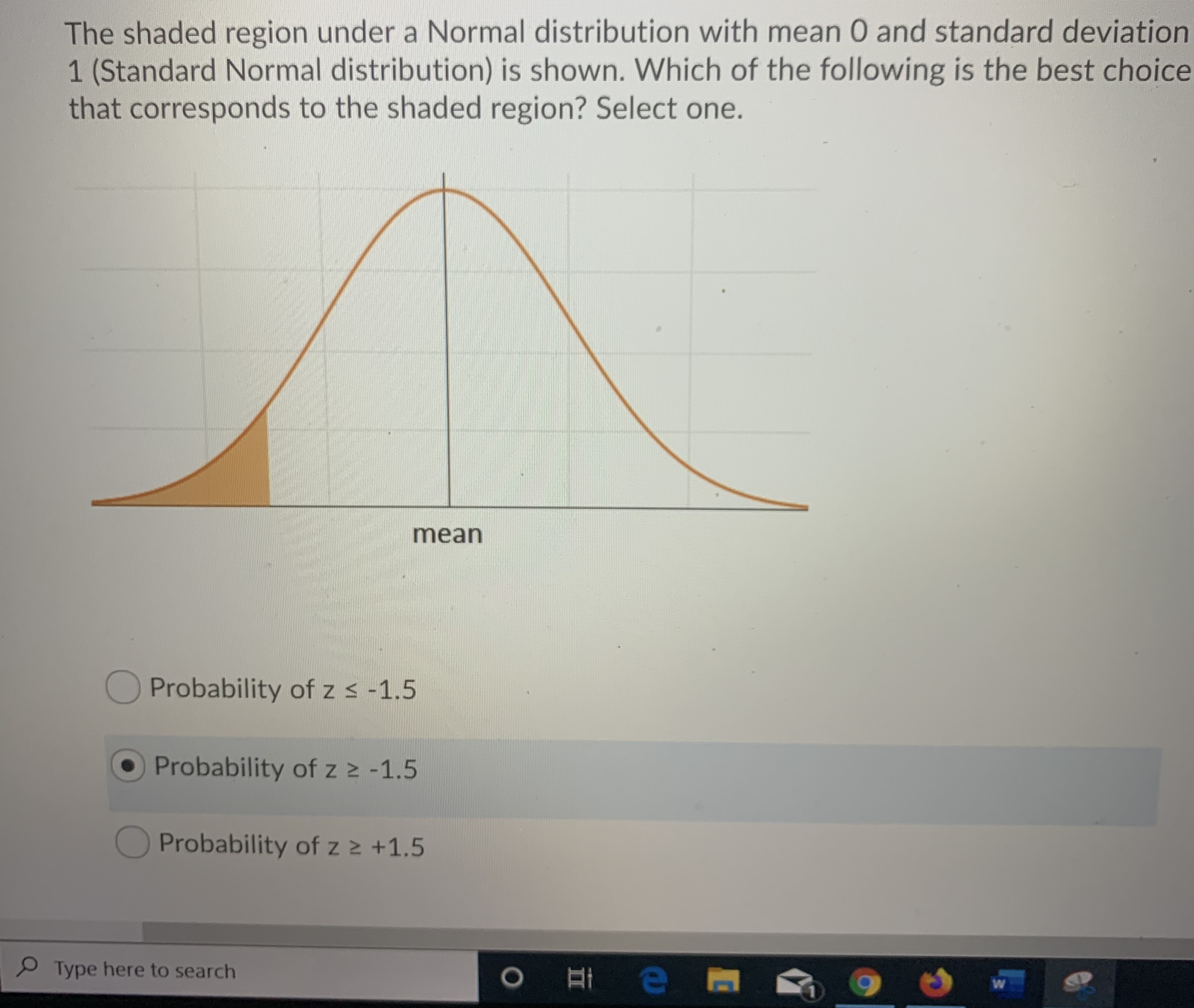# The shaded region under a Normal distribution with mean 0 and standard deviation1 (Standard Normal distribution) is shown. Which of the following is the best choicethat corresponds to the shaded region? Select one.meanProbability of z s -1.5Probability of z2 -1.5Probability of z z +1.5Type here to searchO e

Question
1 viewshelp_outlineImage TranscriptioncloseThe shaded region under a Normal distribution with mean 0 and standard deviation 1 (Standard Normal distribution) is shown. Which of the following is the best choice that corresponds to the shaded region? Select one. mean Probability of z s -1.5 Probability of z2 -1.5 Probability of z z +1.5 Type here to search O e fullscreen
check_circle

Step 1

Standard normal distribution:

The standard normal distribution is a special case of normal distribution, in which the mean of the distribution ...

### Want to see the full answer?

See Solution

#### Want to see this answer and more?

Solutions are written by subject experts who are available 24/7. Questions are typically answered within 1 hour.*

See Solution
*Response times may vary by subject and question.
Tagged in

### Statistics